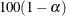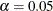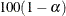The SURVEYREG Procedure

OUTPUT Statement

• OUTPUT <OUT=SAS-data-set> <keyword<=variable-name> …keyword<=variable-name>></ option>;

The OUTPUT statement creates a new SAS data set that contains all the variables in the input data set and, optionally, the estimated linear predictors and their standard error estimates, the residuals from the linear regression, and the confidence limits for the predictors.

You can specify the following options in the OUTPUT statement:

OUT=SAS-data-set

gives the name of the new output data set. By default, the procedure uses the DATAn convention to name the new data set.

keyword <=variable-name>

specifies the statistics to include in the output data set and names the new variables that contain the statistics. You can specify a keyword for each desired statistic (see the following list of keywords). Optionally, you can name a statistic by providing a variable name followed an equal sign to contain the statistic. For example,

output out=myOutDataSet p=myPredictor;


creates a SAS data set myOutDataSet that contains the predicted values in the variable myPredictor.

The keywords allowed and the statistics they represent are as follows:

LCLM | L

lower bound of a% confidence interval for the expected value (mean) of the predicted value. Thelevel is equal to the value of the ALPHA= option in the OUTPUT statement or, if this option is not specified, to the ALPHA= option in the PROC SURVEYREG statement. If neither of these options is set, thenby default, resulting in the lower bound for a 95% confidence interval. If no variable name is given for this keyword, the default variable name is _LCLM_.

PREDICTED | PRED | P

predicted values. If no variable name is given for this keyword, the default variable name is _PREDICTED_.

RESIDUAL | R

residuals, calculated as ACTUAL – PREDICTED. If no variable name is given for this keyword, the default variable name is _RESIDUAL_.

STDP | STD

standard error of the mean predicted value. If no variable name is given for this keyword, the default variable name is _STD_.

UCLM | U

upper bound of a% confidence interval for the expected value (mean) of the predicted value. Thelevel is equal to the value of the ALPHA= option in the OUTPUT statement or, if this option is not specified, to the ALPHA= option in the PROC SURVEYREG statement. If neither of these options is set, thenby default, resulting in the upper bound for a 95% confidence interval. If no variable name is given for this keyword, the default variable name is _UCLM_.

The following option is available in the OUTPUT statement and is specified after a slash (/):

ALPHA=specifies the level of significancefor% confidence intervals. By default,is equal to the value of the ALPHA= option in the PROC SURVEYREG statement or 0.05 if that option is not specified. You can use values between 0 and 1.